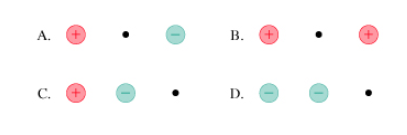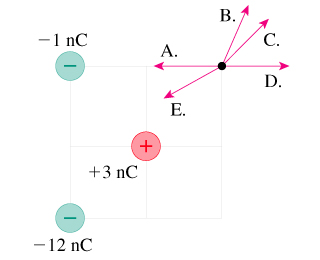# Problem: 1. In which case does the electric field at the dot have the largest magnitude? A, B, C, or D2. Three point charges are arranged as shown in the figure below.Which arrow best represents the direction of the electric field vector at the position of the dot? A, B, C, D, or E

###### FREE Expert Solution

1.

Electric field:

$\overline{){\mathbf{E}}{\mathbf{=}}\frac{\mathbf{k}\mathbf{q}}{{\mathbf{r}}^{\mathbf{2}}}}$

The electric field is proportional to q and inversely proportional to r.

79% (373 ratings)###### Problem Details

1. In which case does the electric field at the dot have the largest magnitude? A, B, C, or D2. Three point charges are arranged as shown in the figure below.Which arrow best represents the direction of the electric field vector at the position of the dot? A, B, C, D, or E